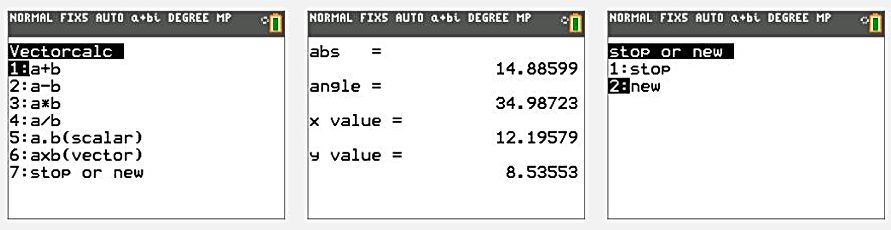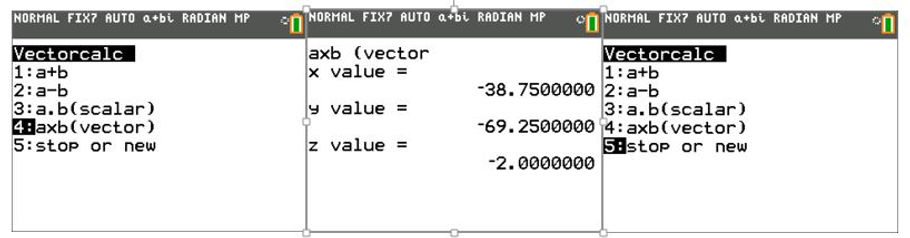naver-site-verification: naver47238a1b6bfbb19a2fd4b619734fa9a6.html
top of page

# Vector calculator 2D and 3D with Ti84 plus

## Calculating the sum, difference, dot and cross product of vectors 2D

A special Ti84 Basic program is available for 2D and 3D vector calculations, such as dot or scalar product and cross or vector product.

Special attention should be paid to the following menus:

nr 3 : a*b = the vector with a length abs(a).abs(b) and an angle φa+φb (used with complex numbers)

nr 4 : a/b = the vector with a length abs(a)/abs(b) and an angle φa- φb (used with complex numbers)

nr 5 : a.b = the dot or the scalar product =abs(a).abs(b)cos(φa-φb) (used in physics)

nr 6 : axb = the cross or vector product = ax*by-ay*bx with a resulting vector perpendicular to both vector a and vector b (in the z-direction if a and b are in the x and y direction)

### Several examples of the vector calculator 2D## Calculating the sum, difference, dot and cross product of 3D vectors

A special Ti84 Basic program has been written for 3D vector calculations.

Special attention for the next menus:

nr 1 : a+b = the sum of vectors

nr 2 : a-b  = the difference of vectors

nr 3 : a.b  = the dot or scalar product

nr 4 : axb = the cross or vector product